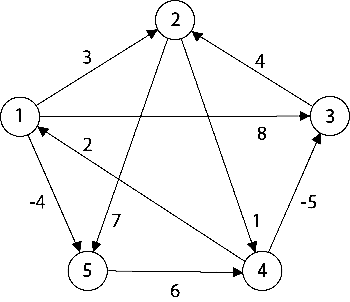The problem is to find the shortest distances between every pair of vertices in a given edge-weighted directed Graph. The Graph is represented as Adjacency Matrix, and the Matrix denotes the weight of the edges (if it exists) else INF (1e7).Input:

The first line of input contains an integer T denoting the no of test cases. Then T test cases follow. The first line of each test case contains an integer V denoting the size of the adjacency matrix. The next V lines contain V space-separated values of the matrix (graph). All input will be an integer type.

Output:

For each test, case output will be V*V space-separated integers where the i-jth integer denotes the shortest distance of the ith vertex from the jth vertex. For INT_MAX integers output INF.

This problem has been previously asked in Samsung.

The Floyd Warshall algorithm, also known as All Pair Shortest Path Algorithm, finds for all the vertices, the minimum cost of going from any one vertex to any other vertex. We do this by checking if there is a path via a particular vertex between two vertices, such that the cost of going via that path is smaller than the current cost of going from one vertex to another.

For example -

Suppose there are two vertices A and C, and the cost of going from one vertex to another is 10. Now let there is another vertex B, such that the cost of going from A to B is 2 and from B to C is 3. So the net cost of going from A to C, via B equals 5, which is smaller, so we update the current cost for A to B, to 5.

Formula for Floyd Warshall Algorithm — if M[a][c] > (M[a][b] + M[b][c]) then M[a][c] = M[a][b] + M[b][c].

The Time Complexity of Floyd Warshall Algorithm is O(n³).

A point to note here is, Floyd Warshall Algorithm does not work for graphs in which there is a negative cycle. In this case, we can use the Bellman-Ford Algorithm, to solve our problem.

The below-given solution is in C programming language.

```#include<stdio.h>

void allPairShortestPath(int M, int v){
int a,b,c;
for(a = 0 ; a < v ; a++){
for(b = 0 ; b < v ; b++){
for(c = 0 ; c < v ; c++){
if(M[b][c] > (M[b][a] + M[a][c]) && (b != a && c != a) && (b != c)){
M[b][c] = M[b][a] + M[a][c];
}
}
}
}

for(b = 0 ; b < v ; b++){
for(c = 0 ; c < v ; c++){
if(M[b][c] == pow(10,7)){
printf("INF ");
}
else{
printf("%d ", M[b][c]);
}
}
printf("\n");
}
}

void main(){
int M;
int a,b,v,t;
scanf("%d", &t);
while(t > 0){
scanf("%d", &v);
for(a = 0 ; a < v ; a++){
for(b = 0 ; b < v ; b++){
scanf("%d",&M[a][b]);
}
}
allPairShortestPath(M, v);
t--;
}
}
```